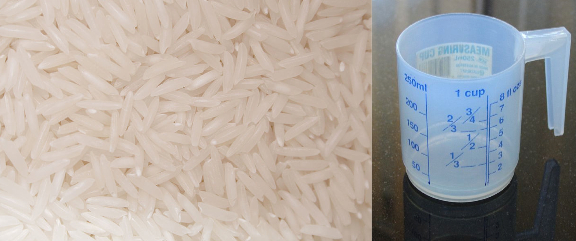# How much rice and how much water?

Posted by: Gary Ernest Davis on: November 28, 2010

The instructions on the rice packet say add$1\frac{1}{2}$ cups of water to every cup of rice.Linda found that this was too much rice, and wanted to reduce the amount.

She came to with the fraction problem: if I only want$\frac{2}{3}$ of a cup of rice, how much water do I need?

I relied: “1 cup.”

She wanted to know how I figured that.

I pointed out that a helpful way to think about the ratio of water to rice is 3 lots – whatever a “lot” is – of water to 2 lots of rice.

So,$\frac{2}{3}$ of a cup of rice is 2 lots of$\frac{1}{3}$ of a cup of rice, so we will need 3 lots of$\frac{1}{3}$ of a cup of water – that is, 1 cup of water.

“OK”, she said, “but say I want$\frac{1}{3}$ of a cup of rice. That might be enough for me. How much water do I need then?”

“Half a cup” I replied.

“Wait”, she said:”How did you get that?”

Because it’s 3 lots of water for every 2 lots of rice, ask yourself what$\frac{1}{3}$ of a cup is 2 lots of.Â  Answer:$\frac{1}{6}$ of a cup. So we need 3 lots of$\frac{1}{6}$ of a cup of water – that is$\frac{1}{2}$ a cup of water.

For more on thinking about fractions see here and also here.

### 4 Responses to "How much rice and how much water?"The proportional thinking is the easy part, especially when your ratios are based on simple whole numbers. You know of a ration for rice to water. You can estimate with it and even imagine the numbers visually, aside from the symbols for ratio of proportion.

Printed cooking directions for a rice package could be wrong in the other direction also. No doubt, your formula would work just as well as a remedy.After getting the answer of “1 cup” for water to be added to 2/3 cup of rice, it’s a roundabout way to repeat “it’s 3 lots of water for every 2 lots of rice …” in order to get the answer for water to be added to 1/3 cup of rice. Just divide the previous answer by 2 to get “half a cup.”Thanks for the comment, and – yes, of course, Yet I think I did not accurately convey the overall confusion my wife had with fractions, not being sure what to do arithmetically, a confusion experienced by many adults who were taught fractions instrumentally. So I wanted to stress the fundamental relationship of 3 for every 2 which is the basis for all these calculations. I was not looking for the quickest, shortest answer, but rather trying to reinforce a basic principle. In fact I did not work out the last piece as I wrote – I mentally thought 3 for every 2, so 3 lots of 1/3 cup is 1 cup, which is 2 lots of 1/2 cup. That’s actually how I did it.I see what you wanted to stress.